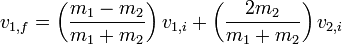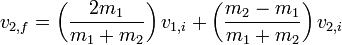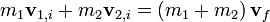# Did I do this right?

## Homework Statement

I was wondering if anybody might help me on this UNLESS i did this correct, but I'm not totally sure.

Well here's the question.

Jorge and Elizabeth are both going to the Antique Road show on PBS. Jorge pushes an antique desk weighing 300kg on a car to the right, while Elizabeth pushes a similar cart to the left with a dresser weighing 225kg. The mass of each cart is 500kg and Jorge pushes his with a speed of 3.0m/s and Elizabeth at a speed of 4.0m/s. If the cars collide and stick together then what is their final velocity and which direction are they headed?

KE=1/2*m*v^2
P=mv

## The Attempt at a Solution

Car 1
500kg+300kg=800kg
3.0 m/s to the left

KE=(.5)mv^2
KE=(.5)(800)(3.0)^2
KE=3600

Car 2
225kg+500kg=725kg
4 m/s to the right

KE=(.5)mv^2
KE=(.5)(725)(4.0m/s)^2
KE=5800

5800-3600=2200

KE=(.5)mv^2
2200=(.5)(mass of Car1 and 2, 1525kg)( v )^2

Solved using calculator

V=1.6986m/s to the right

Am I right?

Last edited:

Delphi51
Homework Helper
Looks like you are using conservation of kinetic energy when you should be using conservation of momentum. Stick-together collisions are not elastic - kinetic energy is lost.

So should I be using P=mv?

jgens
Gold Member
You definately should be using conservation of momentum. In collisions that aren't completely elastic (this one's not as the carts stick together) kinetic energy is not conserved as some energy is lost to heat.

What is conservation of momentum?

jgens
Gold Member
The law of conservation of momentum states that if no net external force acts on a system that the initial and final momentum of the system must be the same; hence, Pi = Pf

So i should use theseIf so, which one?

i got 3.95082 with the first and
2.95082 with the second

Delphi51
Homework Helper
Then put an mv on each side for each object that is moving.
mv + mv = mv (where all the m's and v's are different and perhaps should be numbered)
Put in the numbers and solve for the one unknown speed.

so is it
((M1*V1)+(M2*V2))/(M1+M2)
that way velocity is isolated?

Now I got -.000215

I'm moving on to the next question now

jgens
Gold Member
Cooper43, the equations you posted only apply in the case of a completely elastic collision. The scenario you've described appears to be completely inelastic.

Last edited:
I (think I) FOUNT IT!!!Last edited by a moderator:
jgens
Gold Member
Yes, that equation will suffice for the scenario described in the problem.

(800) (3) + (725) (-4) = (1525)V

V = -0.328 m/s?

they move to the left?

jgens
Gold Member
That's what I got. They move left with a velocity of v = 0.33 m/s. Good job!

Yes, finally. Thanks jgens :)

jgens
Gold Member
You're welcome!